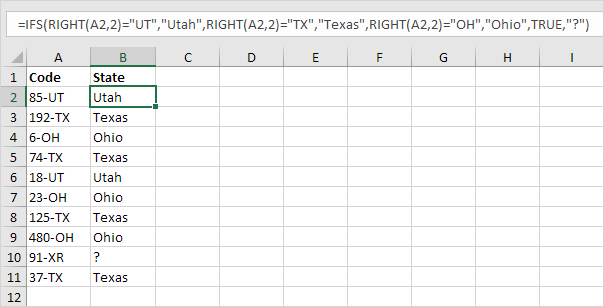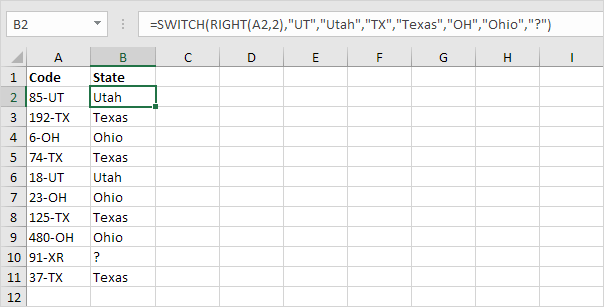# Switch

This example teaches you how to use the SWITCH function in Excel 2016 or later instead of the IFS function.

1a. For example, the IFS function below finds the correct states.Explanation: cell A2 contains the string 85-UT. The RIGHT function extracts the 2 rightmost characters from this string (UT). As a result, the IFS function returns the correct state (Utah). If the 2 rightmost characters are not equal to UT, TX or OH, the IFS function returns a question mark. Instead of TRUE, you can also use 1=1 or something else that is always TRUE.

1b. The SWITCH function below produces the exact same result but is much easier to read.Explanation: if the first argument (RIGHT(A2,2) in this example) equals UT, the SWITCH function returns Utah. If TX, Texas. If OH, Ohio. The last argument (a question mark in this example) is always the default value (if there’s no match).

2. Why not always use the SWITCH function in Excel? There are many examples where you cannot use the SWITCH function instead of the IFS function.Explanation: because we use”<” and “>=” symbols in this IFS function, we cannot use the SWITCH function.

Previous articleShade Alternate Rows in Excel
Next articleTax Rates in Excel